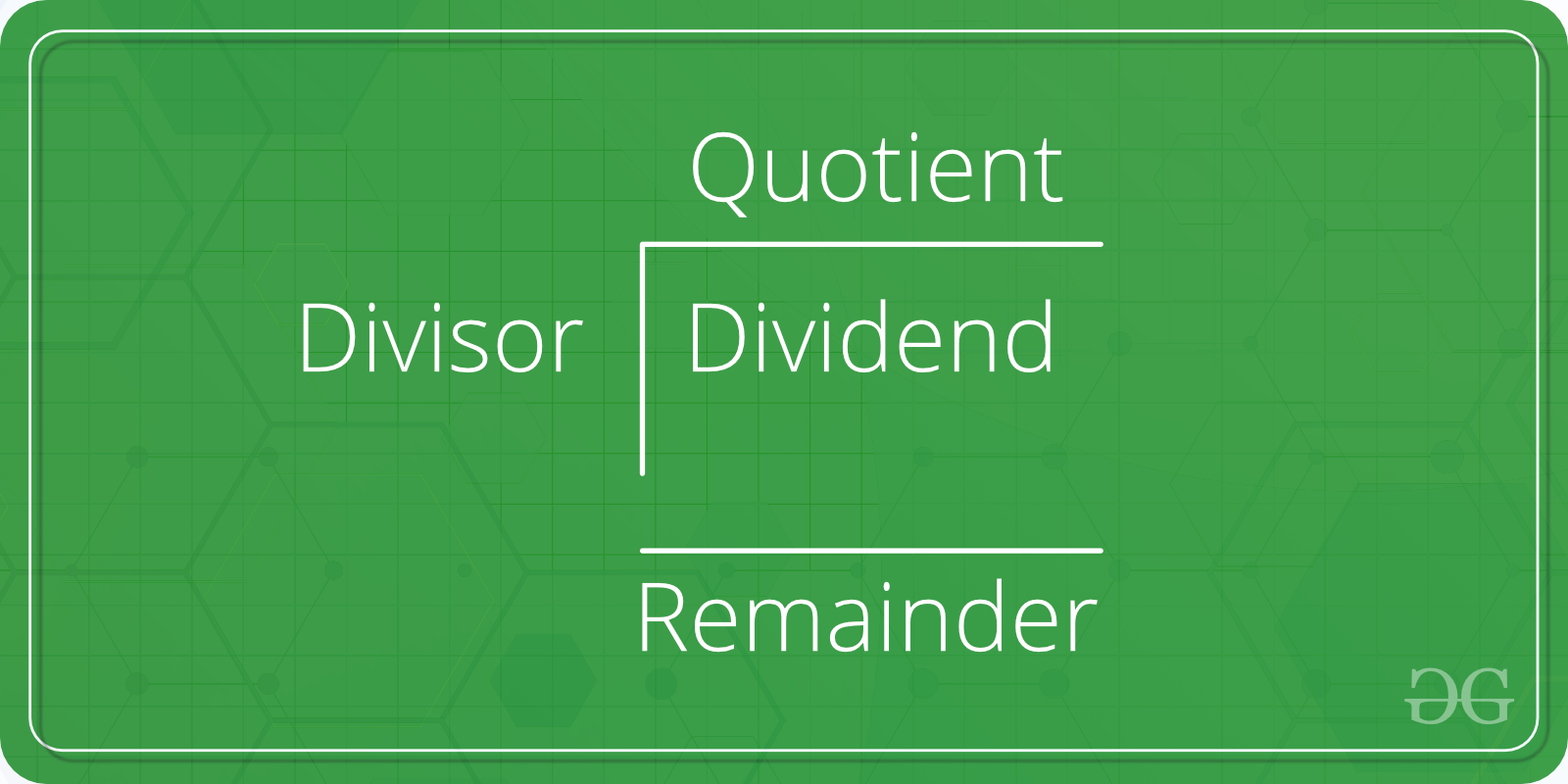# C Program to Compute Quotient and Remainder

Given two numbers A and B. The task is to write a program to find the quotient and remainder of these two numbers when A is divided by B.Examples:

```Input: A = 2, B = 6
Output: Quotient = 0, Remainder = 2

Input: A = 17, B = 5
Output: Quotient = 3, Remainder = 2
```

## Recommended: Please try your approach on {IDE} first, before moving on to the solution.

In the below program, to find the quotient and remainder of the two numbers, the user is first asked to enter two numbers. The inputs are scanned using the scanf() function and stored in the variablesand. Then, the variablesandare divided using the arithmetic operatorto get the quotient as result stored in the variable quotient; and using the arithmetic operator % to get the remainder as result stored in the variable remainder.

Below is the programs to find the quotient and remainder of two numbers:

## C

 `// C program to find quotient ` `// and remainder of two numbers ` ` `  `#include ` ` `  `int` `main() ` `{ ` `    ``int` `A, B, quotient = 0, remainder = 0; ` ` `  `    ``// Ask user to enter the two numbers ` `    ``printf``(``"Enter two numbers A and B : \n"``); ` ` `  `    ``// Read two numbers from the user || A = 17, B = 5 ` `    ``scanf``(``"%d%d"``, &A, &B); ` ` `  `    ``// Calclulate the quotient of A and B using '/' operator ` `    ``quotient = A / B; ` ` `  `    ``// Calclulate the remainder of A and B using '%' operator ` `    ``remainder = A % B; ` ` `  `    ``// Print the result ` `    ``printf``(``"Quotient when A/B is: %d\n"``, quotient); ` `    ``printf``(``"Remainder when A/B is: %d"``, remainder); ` ` `  `    ``return` `0; ` `} `

## Java

 `// java program to find quotient ` `// and remainder of two numbers ` ` `  ` `  `import` `java.io.*; ` `import` `java.util.Scanner; ` ` `  `class` `GFG { ` `  `  ` `  `    ``public` `static` `void` `main (String[] args) { ` `         ``Scanner input = ``new` `Scanner(System.in); ` `    ``int` `A = input.nextInt(); ` `    ``int` `B= input.nextInt(); ` `    ``int`  `    ``quotient = ``0``, remainder = ``0``; ` ` `  `    ``// Ask user to enter the two numbers ` `    ``System.out.println(``"Enter two numbers A and B : "``+``" "``+ A+``" "``+ B); ` ` `  `    ``// Read two numbers from the user || A = 17, B = 5 ` `     `  ` `  ` `  `    ``// Calclulate the quotient of A and B using '/' operator ` `    ``quotient = A / B; ` ` `  `    ``// Calclulate the remainder of A and B using '%' operator ` `    ``remainder = A % B; ` ` `  `    ``// Print the result ` `    ``System.out.println(``"Quotient when A/B is: "``+ quotient); ` `    ``System.out.println(``"Remainder when A/B is: "``+ remainder); ` `    ``} ` `} ` `//this code is contributed by anuj_67.. `

## Python3

 `# Python3 program to find quotient  ` `# and remainder of two numbers ` ` `  `if` `__name__``=``=``'__main__'``: ` `     `  `    ``quotient ``=` `0` `    ``remainder ``=` `0` ` `  ` `  `#Read two numbers from the user || A = 17, B = 5 ` `    ``A, B ``=` `[``int``(x) ``for` `x ``in` `input``().split()] ` ` `  `#Calclulate the quotient of A and B using '/' operator ` `    ``quotient ``=` `int``(A ``/` `B) ` ` `  `#Calclulate the remainder of A and B using '%' operator ` `    ``remainder ``=` `A ``%` `B ` ` `  `#Print the result ` `    ``print``(``"Quotient when A/B is:"``, quotient) ` `    ``print``(``"Remainder when A/B is:"``, remainder) ` ` `  `#this code is contributed by Shashank_Sharma `

Output:

```Enter two numbers A and B : 17 5
Quotient when A/B is: 3
Remainder when A/B is: 2
```

Attention reader! Don’t stop learning now. Get hold of all the important DSA concepts with the DSA Self Paced Course at a student-friendly price and become industry ready.

My Personal Notes arrow_drop_upCheck out this Author's contributed articles.

If you like GeeksforGeeks and would like to contribute, you can also write an article using contribute.geeksforgeeks.org or mail your article to contribute@geeksforgeeks.org. See your article appearing on the GeeksforGeeks main page and help other Geeks.

Please Improve this article if you find anything incorrect by clicking on the "Improve Article" button below.

Improved By : Shashank_Sharma, vt_m